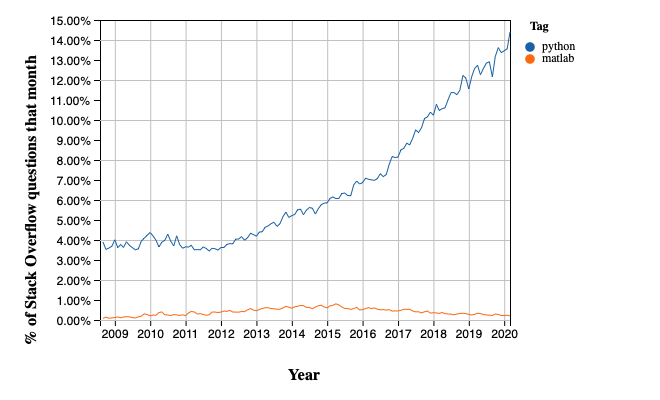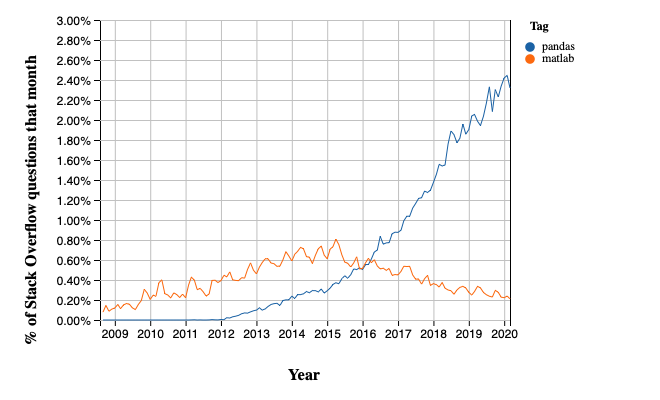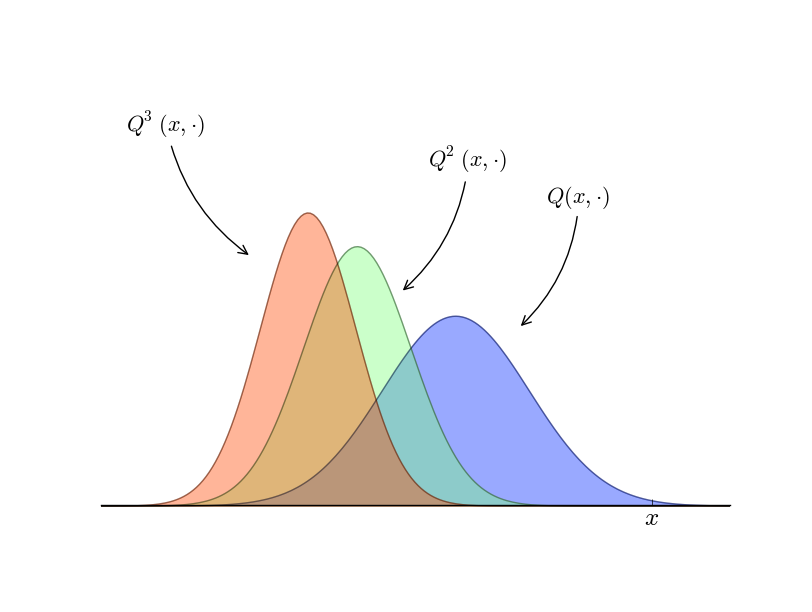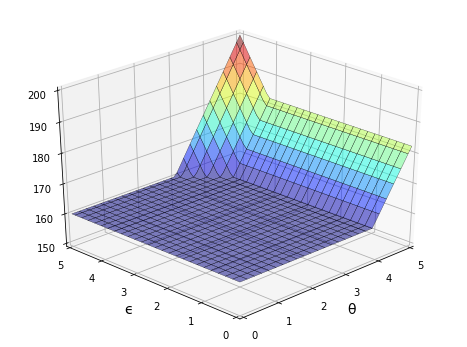# About Python¶

## Contents¶

“Python has gotten sufficiently weapons grade that we don’t descend into R anymore. Sorry, R people. I used to be one of you but we no longer descend into R.” – Chris Wiggins

## Overview¶

In this lecture we will

• outline what Python is
• showcase some of its abilities
• compare it to some other languages.

At this stage, it’s not our intention that you try to replicate all you see.

We will work through what follows at a slow pace later in the lecture series.

Our only objective for this lecture is to give you some feel of what Python is, and what it can do.

## What’s Python?¶

Python is a general-purpose programming language conceived in 1989 by Dutch programmer Guido van Rossum.

Python is free and open source, with development coordinated through the Python Software Foundation.

Python has experienced rapid adoption in the last decade and is now one of the most popular programming languages.

### Common Uses¶

Python is a general-purpose language used in almost all application domains such as

• communications
• web development
• CGI and graphical user interfaces
• game development
• multimedia, data processing, security, etc., etc., etc.

Used extensively by Internet services and high tech companies including

Python is very beginner-friendly and is often used to teach computer science and programming.

For reasons we will discuss, Python is particularly popular within the scientific community with users including NASA, CERN and practically all branches of academia.

It is also replacing familiar tools like Excel in the fields of finance and banking.

### Relative Popularity¶

The following chart, produced using Stack Overflow Trends, shows one measure of the relative popularity of PythonThe figure indicates not only that Python is widely used but also that adoption of Python has accelerated significantly since 2012.

We suspect this is driven at least in part by uptake in the scientific domain, particularly in rapidly growing fields like data science.

For example, the popularity of pandas, a library for data analysis with Python has exploded, as seen here.

(The corresponding time path for MATLAB is shown for comparison)Note that pandas takes off in 2012, which is the same year that we see Python’s popularity begin to spike in the first figure.

Overall, it’s clear that

### Features¶

Python is a high-level language suitable for rapid development.

It has a relatively small core language supported by many libraries.

Other features of Python:

• multiple programming styles are supported (procedural, object-oriented, functional, etc.)
• it is interpreted rather than compiled.

### Syntax and Design¶

One nice feature of Python is its elegant syntax — we’ll see many examples later on.

Elegant code might sound superfluous but in fact it’s highly beneficial because it makes the syntax easy to read and easy to remember.

Remembering how to read from files, sort dictionaries and other such routine tasks means that you don’t need to break your flow in order to hunt down correct syntax.

Closely related to elegant syntax is an elegant design.

Features like iterators, generators, decorators and list comprehensions make Python highly expressive, allowing you to get more done with less code.

Namespaces improve productivity by cutting down on bugs and syntax errors.

## Scientific Programming¶

Python has become one of the core languages of scientific computing.

It’s either the dominant player or a major player in

Its popularity in economics is also beginning to rise.

This section briefly showcases some examples of Python for scientific programming.

• All of these topics will be covered in detail later on.

### Numerical Programming¶

Fundamental matrix and array processing capabilities are provided by the excellent NumPy library.

NumPy provides the basic array data type plus some simple processing operations.

For example, let’s build some arrays

In [ ]:
import numpy as np                     # Load the library

a = np.linspace(-np.pi, np.pi, 100)    # Create even grid from -π to π
b = np.cos(a)                          # Apply cosine to each element of a
c = np.sin(a)                          # Apply sin to each element of a


Now let’s take the inner product

In [ ]:
b @ c


The number you see here might vary slightly but it’s essentially zero.

(For older versions of Python and NumPy you need to use the np.dot function)

The SciPy library is built on top of NumPy and provides additional functionality.

For example, let’s calculate $\int_{-2}^2 \phi(z) dz$ where $\phi$ is the standard normal density.

In [ ]:
from scipy.stats import norm
from scipy.integrate import quad

ϕ = norm()
value, error = quad(ϕ.pdf, -2, 2)  # Integrate using Gaussian quadrature
value


SciPy includes many of the standard routines used in

See them all here.

### Graphics¶

The most popular and comprehensive Python library for creating figures and graphs is Matplotlib, with functionality including

• plots, histograms, contour images, 3D graphs, bar charts etc.
• output in many formats (PDF, PNG, EPS, etc.)
• LaTeX integration

Example 2D plot with embedded LaTeX annotationsExample contour plotExample 3D plotMore examples can be found in the Matplotlib thumbnail gallery.

Other graphics libraries include

### Symbolic Algebra¶

It’s useful to be able to manipulate symbolic expressions, as in Mathematica or Maple.

The SymPy library provides this functionality from within the Python shell.

In [ ]:
from sympy import Symbol

x, y = Symbol('x'), Symbol('y')  # Treat 'x' and 'y' as algebraic symbols
x + x + x + y


We can manipulate expressions

In [ ]:
expression = (x + y)**2
expression.expand()


solve polynomials

In [ ]:
from sympy import solve

solve(x**2 + x + 2)


and calculate limits, derivatives and integrals

In [ ]:
from sympy import limit, sin, diff

limit(1 / x, x, 0)

In [ ]:
limit(sin(x) / x, x, 0)

In [ ]:
diff(sin(x), x)


The beauty of importing this functionality into Python is that we are working within a fully fledged programming language.

We can easily create tables of derivatives, generate LaTeX output, add that output to figures and so on.

### Statistics¶

Python’s data manipulation and statistics libraries have improved rapidly over the last few years.

#### Pandas¶

One of the most popular libraries for working with data is pandas.

Pandas is fast, efficient, flexible and well designed.

Here’s a simple example, using some dummy data generated with Numpy’s excellent random functionality.

In [ ]:
import pandas as pd
np.random.seed(1234)

data = np.random.randn(5, 2)  # 5x2 matrix of N(0, 1) random draws
dates = pd.date_range('28/12/2010', periods=5)

df = pd.DataFrame(data, columns=('price', 'weight'), index=dates)
print(df)

In [ ]:
df.mean()


#### Other Useful Statistics Libraries¶

• scikit-learn — machine learning in Python (sponsored by Google, among others)

• pyMC — for Bayesian data analysis

### Networks and Graphs¶

Python has many libraries for studying graphs.

One well-known example is NetworkX. Its features include, among many other things:

• standard graph algorithms for analyzing networks
• plotting routines

Here’s some example code that generates and plots a random graph, with node color determined by shortest path length from a central node.

In [ ]:
%matplotlib inline
import networkx as nx
import matplotlib.pyplot as plt
plt.rcParams['figure.figsize'] = (10,6)
np.random.seed(1234)

# Generate a random graph
p = dict((i, (np.random.uniform(0, 1), np.random.uniform(0, 1)))
for i in range(200))
g = nx.random_geometric_graph(200, 0.12, pos=p)
pos = nx.get_node_attributes(g, 'pos')

# Find node nearest the center point (0.5, 0.5)
dists = [(x - 0.5)**2 + (y - 0.5)**2 for x, y in list(pos.values())]
ncenter = np.argmin(dists)

# Plot graph, coloring by path length from central node
p = nx.single_source_shortest_path_length(g, ncenter)
plt.figure()
nx.draw_networkx_edges(g, pos, alpha=0.4)
nx.draw_networkx_nodes(g,
pos,
nodelist=list(p.keys()),
node_size=120, alpha=0.5,
node_color=list(p.values()),
cmap=plt.cm.jet_r)
plt.show()


### Cloud Computing¶

Running your Python code on massive servers in the cloud is becoming easier and easier.

A nice example is Anaconda Enterprise.

See also

### Parallel Processing¶

Apart from the cloud computing options listed above, you might like to consider

### Other Developments¶

There are many other interesting developments with scientific programming in Python.

Some representative examples include

• Jupyter — Python in your browser with interactive code cells, embedded images and other useful features.

• Numba — Make Python run at the same speed as native machine code!

• Blaze — a generalization of NumPy.

• CVXPY — convex optimization in Python.